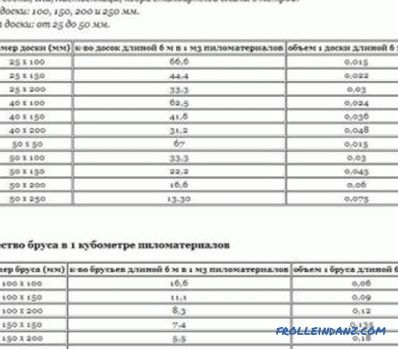# How to calculate the cubic capacity of round wood on the basis of tables

The log trunk represents a certain volume of lumber, the unit of measurement of which is the cube. This parameter is used when erecting wooden houses to calculate the required volume of roundwood. The determined indicator is very important when calculating the payment for the sold lumber for the construction of the house, since it is necessary to know the cost of each cube. m tree.The round wood is very convenient and practical for building houses, baths and other residential and non-residential premises.

## Method of correctly counting lumber

There are various options for calculating the cubic capacity of a log, which is a circular cylindrical lumber. This estimate is indicative. The methods used provide the initial geometric parameters on the basis of which the calculations are made.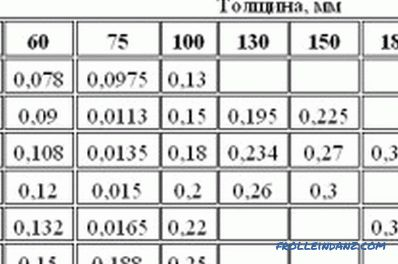Table for calculating the cubic capacity of a log and bar.

Industrial harvesting of lumber is associated with quite complex calculations that are carried out in order to find out the volume of the forest. For accurate calculations, a special truncated cone formula is used. It is based on the determination of the basic diameters of each of the two saw cuts and the length of the logs.

In practice, this complex method is rarely used, since the global standards defining the cubic capacity of round wood require the use of special tables. The calculation of the volume of the forest when building a house in this way is not particularly difficult. The result is quite accurate, and the calculation is based on the value of the volume of the material in the form of a parallelepiped and the average value of the thickness of the log cut in its upper part.

### How to calculate the cubic capacity of a log in a single variant correctly?

Until now, the calculation of the cubature of a single tree was determined by multiplying its length by the arithmetic average of the total area of ​​all saw cuts. This method involved the use of a special measuring device, which resembled a caliper.

Correctly calculate the cubic capacity of roundwood, taking the log unit as the basis, without preliminary measurements can be made with the following tools:

1. Roulettes.
2. Calculated tables with indication of final cubature.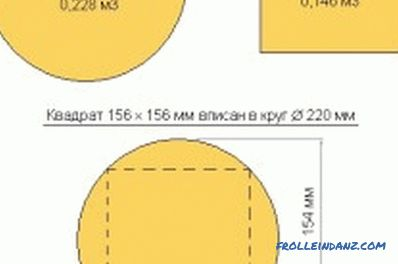Types of lumber and their volume.

Before calculating the cubic capacity of a log, the size of its diameter in the middle part was increased three times, getting a certain value of the cross-sectional area. It was required to multiply by the length of the workpiece, in order to get the value of the volume of the material. This method was not accurate because the thickness of the bark of the tree was not taken into account.

The value of Pi in the formula of a circle was applied with a very large deviation, therefore the distorted form of the formula did not allow making accurate calculations without errors. In practice, the following formula is used: the diameter of a round tree divided by 2 is squared, then the result is multiplied by Pi and the size of the tree length.

It is impossible to argue that it is correct to count the volume of the forest for the construction of a house only on the basis of tables, special measurements and relevant formulas. The simplest is the method associated with determining the density of wood species.

If you count the cubic capacity of round wood by measuring the thickness of the wood bark of lumber for building houses, then it is impossible to determine the diameter of the logs without possible errors. Calculated parameters will be inaccurate. To correctly calculate the volume of a single wooden material, make measurements of its length with a tape measure, then measure the diameter of the cut at the top, not taking into account the thickness of the bark.

Next, you should look at a special table in which at the intersection of the rows and columns with the given lengths of the tree, as well as its diameter, determine the amount of material volume. This method is reliable. Before calculating the volume of the forest in this way, you should know that it is not perfectly accurate, since it does not take into account the shape of the trunk and the conditions associated with growing forest for the construction of houses. However, these little things are usually ignored.

### How to count the cubic capacity of a round stored forest?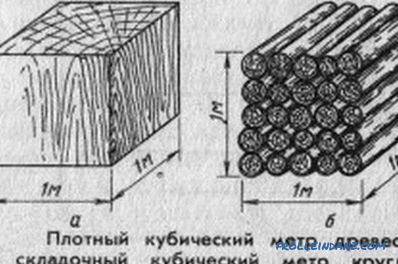Figure 1. Calculation of the cubic capacity of a round stored forest.

If we consider the industrial volumes of logs of round timber, here we use other tables and parameters of a round log, which allow to calculate the cubic capacity. As shown in the figure (IMAGE 1), roundwood in storage conditions has the shape of a parallelepiped in the form of a rectangle.

The method of calculating this volume is simple and well-known from school knowledge. In practice, the use of this method does not give an accurate result, since the dimensions of the voids between individual logs are not taken into account. The dimensions of the voids depend on the diameter of the logs and can be calculated by the mathematical method.Calculate the exact values ​​in the case of inaccurate stacking of log blanks will not work. This procedure is not carried out in the course of the evaluation of the forest stored at the warehouse, as the volume occupied by the round forest at the warehouse itself is estimated.

If we carry out a preliminary weighing of the forest, then it will not be difficult to calculate the cubic capacity, since it is not completely filled with wood material. Reduce the desired value should be using the coefficient of voids. When performing construction calculations, the value of the coefficient of voids is equal to 0.8, which is 20% of the area of ​​space that is occupied by air void, and 80% is the volume of the tree.

The sequence of actions for determining the cubic size is reduced to the following main stages:

1. Measuring the width, length, height of a space that is rectangular.
2. Multiplication between certain quantities to calculate the result of a volume.
3. Multiply the result by a factor of 0.8, which takes into account the number of voids between the logs, which is approximate.
4. Acceptance of the obtained value for effective.

Using this method, the cubic capacity of round wood is calculated, which is heterogeneous in size.

## Making calculations depending on the type of material

To calculate the volume of lumber, divide the mass of wood by its density, it is necessary to take into account the type of wood.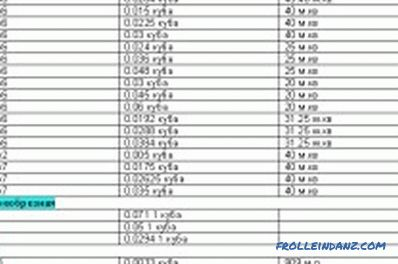Figure 2. Table for calculating the cubic capacity of unedged and edged board, bar, wall paneling, lath, etc.

Received the result will be far from ideal, because the forest may have a different degree of maturity, hence the different deviations in density. The main role belongs to the moisture content of the wood.

For the woodworking industry, concepts related to dense cube are typical. m and folding. The price list for such sawn timber will contain information about the volume in its dense mass; a conversion of the cubic meter into a dense mass will be required. For this purpose, conversion factors are used.

The volume of the coniferous or hardwood forest is calculated by two methods:

1. By measuring all units of the tree.
2. Through the application of a standard, that is, a cubature designed according to GOST.

A cubic table is designed to calculate the volume of edged wood material based on standard tables. In one you can find the volume of a meter of wood, and in the second - units, which allows you to make a calculation of the value of the forest.

The proposed table (IMAGE 2) contains calculations for the cubic capacity of unedged and edged boards, bars, lining, slats, etc. The dimension has its own specifics, that is, the width of any board is equal to half the width of a narrow and wide layers measured in the middle of the board. The calculation of the volume of a round material is made correctly if each log is measured.

•